• 具有对角带宽矩阵的快速准确的最先进的双变量核密度估计器。 核被假定为高斯核。 两个带宽参数都是经过优化选择的使用/假设数据的参数模型或任何“经验法则”。 与许多其他程序不同，这个程序在估计具有广泛分离模式...matlab
• 针对密度峰值聚类算法CFSFDP（clustering by fast search and find of density peaks）计算密度时人为判断截断距离和人工截取簇类中心的缺陷，提出了一种基于非参数核密度估计的密度峰值聚类算法。首先，应用非参数...聚类
• 针对金融资产收益率分布呈现的尖峰、厚尾及有偏的特点，沿袭变换核密度估计的思想，提出一种广义Logistic变换，对变换后的样本应用Beta核密度估计以消除边界偏差....
• 基于边界检测的无线传感器网络 能量密度路由算法的研究，罗建辉，伍仁勇，网络的拓扑识别是无线传感器网络研究中的重要问题，而边界识别是其核心问题。本文在现有的边界识别算法和无线传感器网络经典路由无线传感器网络
• 核心对象：圆内的点(条件：点数量大于等于设定阈值(minPts)的个数)阈值(minPts):圆的半径大小直接密度可达：点p在点q的圆内，且q是核心点，p-q是... 边界点:属于某一个类的非核心点,不能发展下线了离群点：不属于任...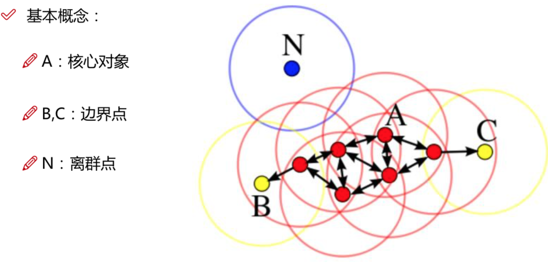核心对象：圆内的点(条件：点数量大于等于设定阈值(minPts)的个数)阈值(minPts):圆的半径大小直接密度可达：点p在点q的圆内，且q是核心点，p-q是直接密度可达密度可达：若有一个点的序列q0、q1、...qk，对任意qi-qi-1是直接密度可达的 ，则称从q0到qk密度可达，这实际上是直接密度可达的“传播”。 边界点:属于某一个类的非核心点,不能发展下线了离群点：不属于任何一个类簇的点参数选择：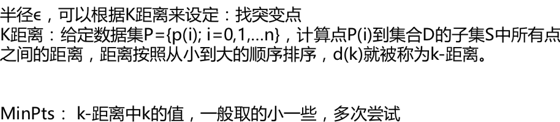优缺点：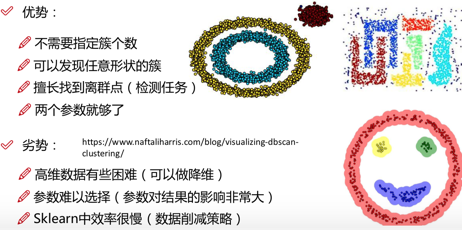转载本博笔记须在文章明显处注明原文的链接和作者信息

转载于:https://www.cnblogs.com/yinghuali/p/9255161.html
展开全文• DBSCAN的核心思想是从某个核心点出发，不断向密度可达的区域扩张，从而得到一个包含核心点和边界点的最大化区域，区域中任意两点密度相连。1、伪代码 算法： DBSCAN 输入： E — 半径 MinPts — 给定点在 E ...
文章目录@[toc]一、DBSCAN聚类概述1、伪代码2、优点：3、缺点：4、与其他聚类算法比较二、sklearn中的DBSCAN聚类算法1、主要函数介绍：最重要的两个参数：其他主要属性：运行式子：2、DBSCAN自编代码3、实战案例：延伸一：DPEAK算法——密度最大值算法延伸二：利用GPS轨迹和DBSCAN推断工作地居住地延伸三：HDBSCAN与Kmeans的聚类的一些纪要
一、DBSCAN聚类概述
基于密度的方法的特点是不依赖于距离，而是依赖于密度，从而克服基于距离的算法只能发现“球形”聚簇的缺点。
DBSCAN的核心思想是从某个核心点出发，不断向密度可达的区域扩张，从而得到一个包含核心点和边界点的最大化区域，区域中任意两点密度相连。
1、伪代码

算法： DBSCAN
输入： E — 半径
MinPts — 给定点在 E 领域内成为核心对象的最小领域点数
D — 集合
输出：目标类簇集合
方法： repeat

  判断输入点是否为核心对象


  找出核心对象的 E 领域中的所有直接密度可达点

util 所有输入点都判断完毕
repeat
针对所有核心对象的 E 领域所有直接密度可达点找到最大密度相连对象集合，
中间涉及到一些密度可达对象的合并。
Util 所有核心对象的 E 领域都遍历完毕
密度：空间中任意一点的密度是以该点为圆心，以EPS为半径的圆区域内包含的点数目
边界点：空间中某一点的密度，如果小于某一点给定的阈值minpts,则称为边界点
噪声点：不属于核心点，也不属于边界点的点，也就是密度为1的点

2、优点：
这类算法能克服基于距离的算法只能发现“类圆形”(凸)的聚类的缺点
可发现任意形状的聚类，且对噪声数据不敏感。
不需要指定类的数目cluster
算法中只有两个参数，扫描半径 (eps)和最小包含点数(min_samples)
3、缺点：
1、计算复杂度，不进行任何优化时，算法的时间复杂度是O(N^{2})，通常可利用R-tree，k-d tree, ball
tree索引来加速计算，将算法的时间复杂度降为O(Nlog(N))。
2、受eps影响较大。在类中的数据分布密度不均匀时，eps较小时，密度小的cluster会被划分成多个性质相似的cluster；eps较大时，会使得距离较近且密度较大的cluster被合并成一个cluster。在高维数据时，因为维数灾难问题，eps的选取比较困难。
3、依赖距离公式的选取，由于维度灾害，距离的度量标准不重要
4、不适合数据集集中密度差异很大的，因为eps和metric选取很困难
4、与其他聚类算法比较
来看两张图：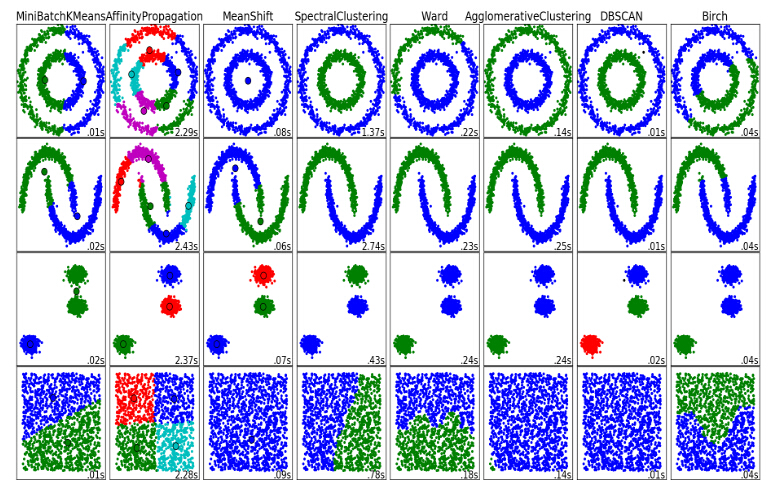DBSCAN可以较快、较有效的聚类出来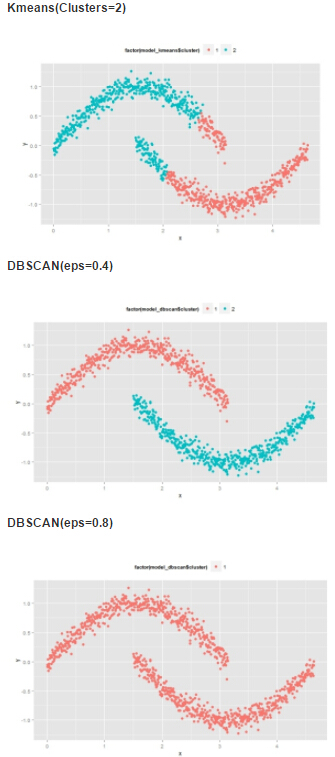eps的取值对聚类效果的影响很大。
.
二、sklearn中的DBSCAN聚类算法
1、主要函数介绍：
DBSCAN(eps=0.5, min_samples=5, metric='euclidean', algorithm='auto', leaf_size=30, p=None, n_jobs=1)

最重要的两个参数：
eps:两个样本之间的最大距离，即扫描半径
min_samples ：作为核心点的话邻域(即以其为圆心，eps为半径的圆，含圆上的点)中的最小样本数(包括点本身)。
其他参数：
metric ：度量方式，默认为欧式距离，还有metric=‘precomputed’（稀疏半径邻域图）
algorithm：近邻算法求解方式，有四种：‘auto’, ‘ball_tree’, ‘kd_tree’, ‘brute’
leaf_size：叶的大小，在使用BallTree or cKDTree近邻算法时候会需要这个参数
n_jobs ：使用CPU格式，-1代表全开
其他主要属性：
core_sample_indices_:核心样本指数。（此参数在代码中有详细的解释）
labels_:数据集中每个点的集合标签给,噪声点标签为-1。
components_ ：核心样本的副本
运行式子：
model = sklearn.cluster.DBSCAN(eps_领域大小圆半径,min_samples_领域内，点的个数的阈值)
model.fit(data) 训练模型
model.fit_predict(data) 模型的预测方法
.
2、DBSCAN自编代码
来源：【挖掘模型】：Python-DBSCAN算法
import numpy
import pandas
import matplotlib.pyplot as plt

#导入数据
data = pandas.read_csv("F:\\python 数据挖掘分析实战\\Data\\data (7).csv")

plt.scatter(
data['x'],
data['y']
)

eps = 0.2;
MinPts = 5;

from sklearn.metrics.pairwise import euclidean_distances

ptses = []
dist = euclidean_distances(data)
for row in dist:
#密度,空间中任意一点的密度是以该点为圆心、以 Eps 为半径的圆区域内包含的点数
density = numpy.sum(row<eps)
pts = 0;
if density>MinPts:
#核心点（Core Points）
#空间中某一点的密度，如果大于某一给定阈值MinPts，则称该为核心点
pts = 1
elif density>1 :
#边界点（Border Points）
#空间中某一点的密度，如果小于某一给定阈值MinPts，则称该为边界点
pts = 2
else:
#噪声点（Noise Points）
#数据集中不属于核心点，也不属于边界点的点，也就是密度值为1的点
pts = 0
ptses.append(pts)

#把噪声点过滤掉，因为噪声点无法聚类，它们独自一类
corePoints = data[pandas.Series(ptses)!=0]

coreDist = euclidean_distances(corePoints)

#首先，把每个点的领域都作为一类
#邻域（Neighborhood）
#空间中任意一点的邻域是以该点为圆心、以 Eps 为半径的圆区域内包含的点集合
cluster = dict();
i = 0;
for row in coreDist:
cluster[i] = numpy.where(row<eps)
i = i + 1

#然后，将有交集的领域，都合并为新的领域
for i in range(len(cluster)):
for j in range(len(cluster)):
if len(set(cluster[j]) & set(cluster[i]))>0 and i!=j:
cluster[i] = list(set(cluster[i]) | set(cluster[j]))
cluster[j] = list();

#最后，找出独立（也就是没有交集）的领域，就是我们最后的聚类的结果了
result = dict();
j = 0
for i in range(len(cluster)):
if len(cluster[i])>0:
result[j] = cluster[i]
j = j + 1

#找出每个点所在领域的序号，作为他们最后聚类的结果标记
for i in range(len(result)):
for j in result[i]:
data.at[j, 'type'] = i

plt.scatter(
data['x'],
data['y'],
c=data['type']
)

3、实战案例：
# DBSCAN clustering algorithm

print(__doc__)

import numpy as np

from sklearn.cluster import DBSCAN
from sklearn import metrics
from sklearn.datasets.samples_generator import make_blobs
from sklearn.preprocessing import StandardScaler

# Generate sample data
centers = [[1, 1], [-1, -1], [1, -1]]
X, labels_true = make_blobs(n_samples=750, centers=centers, cluster_std=0.4,
random_state=0)

X = StandardScaler().fit_transform(X)

# Compute DBSCAN
db = DBSCAN(eps=0.1, min_samples=10).fit(X)
core_samples_mask = np.zeros_like(db.labels_, dtype=bool)
core_samples_mask[db.core_sample_indices_] = True
labels = db.labels_

# Number of clusters in labels, ignoring noise if present.
n_clusters_ = len(set(labels)) - (1 if -1 in labels else 0)

print('Estimated number of clusters: %d' % n_clusters_)
print("Homogeneity: %0.3f" % metrics.homogeneity_score(labels_true, labels))
print("Completeness: %0.3f" % metrics.completeness_score(labels_true, labels))
print("V-measure: %0.3f" % metrics.v_measure_score(labels_true, labels))
print("Adjusted Rand Index: %0.3f"
% metrics.adjusted_rand_score(labels_true, labels))
print("Adjusted Mutual Information: %0.3f"
% metrics.adjusted_mutual_info_score(labels_true, labels))
print("Silhouette Coefficient: %0.3f"
% metrics.silhouette_score(X, labels))

#
import matplotlib.pyplot as plt

# Black removed and is used for noise instead.
unique_labels = set(labels)
colors = [plt.cm.Spectral(each)
for each in np.linspace(0, 1, len(unique_labels))]
for k, col in zip(unique_labels, colors):
if k == -1:
# Black used for noise.
col = [0, 0, 0, 1]

class_member_mask = (labels == k)

xy = X[class_member_mask & core_samples_mask]
plt.plot(xy[:, 0], xy[:, 1], 'o', markerfacecolor=tuple(col),
markeredgecolor='k', markersize=14)

xy = X[class_member_mask & ~core_samples_mask]
plt.plot(xy[:, 0], xy[:, 1], 'o', markerfacecolor=tuple(col),
markeredgecolor='k', markersize=6)

plt.title('Estimated number of clusters: %d' % n_clusters_)
plt.show()

最后的结果：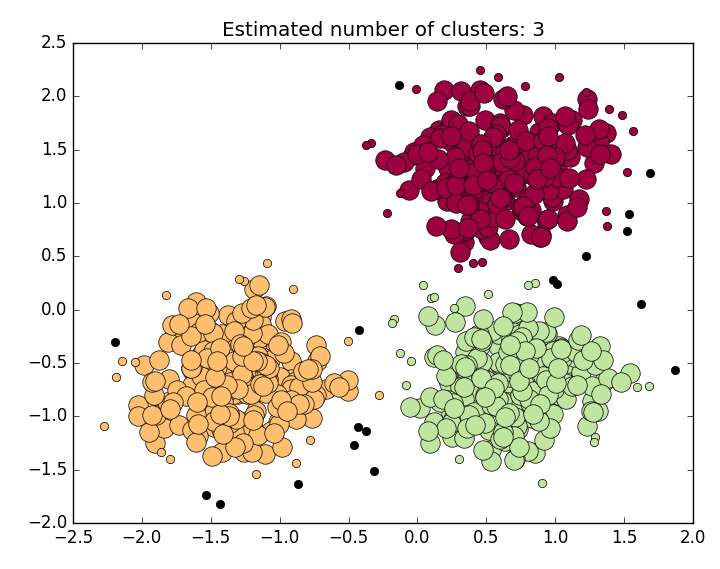.
延伸一：DPEAK算法——密度最大值算法
本节来源：机器学习笔记（九）聚类算法及实践（K-Means,DBSCAN,DPEAK,Spectral_Clustering）、聚类 - 4 - 层次聚类、密度聚类(DBSCAN算法、密度最大值聚类)
密度最大值聚类是一种简洁优美的聚类算法, 可以识别各种形状的类簇, 并且参数很容易确定。用于找聚类中心和异常值的。
用DPEAK算法找到聚类中心之后，在用DBSCAN会更好
（1）我们首先给定一个半径范围r，然后对我们所有的样本，计算它的r邻域内的样本数目记作它的局部密度记作rho
（2）第二步，计算每个样本到密度比它高的点的距离的最小值记作sigma，有了这两个参数就可以进行我们下一步的筛选工作了
具体分成以下四种情况：
1 rho很小，sigma很大。这个样本周围的样本量很小，但是到比它密度大的点的距离还挺远的，这说明啥，它是个远离正常样本的异常值啊，在偏僻的小角落里搞自己的小动作啊，果断踢了它呀。
2 rho很大，sigma也很大。这个样本周围样本量很大，并且要找到比它密度还大的点要好远好远，这说明这个点是被众星环绕的啊，它就是这个簇的王，我们往往把它确定为簇中心。
3 rho很小，sigma也很小。样本周围的样本量很小，但要找到样本密度比它大的点没多远就有，说明这个点是一个处在边缘上的点，往往是一个簇的边界。
4 rho很大，sigma很小。该样本周围的样本量很大，但是密度比它还大的居然也不远，这种情况只会发生在你处在了簇中心的旁边时，很可惜，也许你是这个簇的核心成员，但你做不了这个簇的王。
好的，基于每个样本的rho和sigma，我们大概就能确定它们各自的所扮演的角色了，我们把大反派异常值从样本中剔除，然后把我们找到的rho和sigma都很大的点作为簇中心，再利用K-Means或者DBSCAN算法进行聚类就能得到相对比较好的结果。
延伸二：利用GPS轨迹和DBSCAN推断工作地居住地
来自文章《利用GPS轨迹和DBSCAN推断工作地居住地》
利用移动设备用户的GPS轨迹可以推断用户的工作地和居住地。本文中的数据集来自微软亚洲研究院的GeoLife GPS轨迹数据集
利用DBSCAN（Density-Based Spatial Clustering and Application with Noise）算法对数据集进行聚类。DBSCAN是一种聚类算法，这种算法通常用于对带有噪声的空间数据进行聚类。利用DBSCAN算法可以对GPS轨迹聚成4类。直观上来看，每一个聚类都表示该用户经常到访该区域。因此，可以假设用户的工作地和居住地就在这4个聚类中。
延伸三：HDBSCAN与Kmeans的聚类的一些纪要
HDBSCAN的安装与使用
安装的两种办法：
conda install -c conda-forge hdbscan  # first
pip install hdbscan    # second

应用：
import hdbscan
from sklearn.datasets import make_blobs

data, _ = make_blobs(1000)

clusterer = hdbscan.RobustSingleLinkage(cut=0.125, k=7)
cluster_labels = clusterer.fit_predict(data)
hierarchy = clusterer.cluster_hierarchy_
alt_labels = hierarchy.get_clusters(0.100, 5)
hierarchy.plot()

DBSCAN vs HDBSCAN
HDBSCAN - Hierarchical Density-Based Spatial Clustering of Applications with Noise. Performs DBSCAN over varying epsilon values and integrates the result to find a clustering that gives the best stability over epsilon. This allows HDBSCAN to find clusters of varying densities (unlike DBSCAN), and be more robust to parameter selection.
如果输入数据的变量类型不同，部分是数值型（numerical），部分是分类变量（categorical），需要做特别处理。
方法1是将分类变量转化为数值型，但缺点在于如果使用独热编码（one hot encoding）可能会导致数据维度大幅度上升，如果使用标签编码（label encoding）无法很好的处理数据中的顺序（order）。方法2是对于数值型变量和分类变量分开处理，并将结果结合起来，具体可以参考Python的实现，如K-mode和K-prototype。
输出结果非固定，多次运行结果可能不同。
首先要意识到K-means中是有随机性的，从初始化到收敛结果往往不同。一种看法是强行固定随机性，比如设定sklearn中的random state为固定值。另一种看法是，如果你的K均值结果总在大幅度变化，比如不同簇中的数据量在多次运行中变化很大，那么K均值不适合你的数据，不要试图稳定结果 
运行效率与性能之间的取舍。
但数据量上升到一定程度时，如>10万条数据，那么很多算法都不能使用。最近读到的一篇对比不同算法性能随数据量的变化很有意思 [Benchmarking Performance and Scaling of Python Clustering Algorithms]。在作者的数据集上，当数据量超过一定程度时仅K均值和HDBSCAN可用。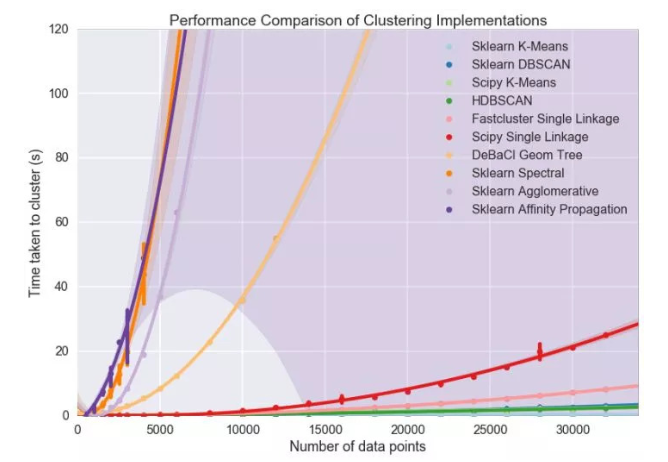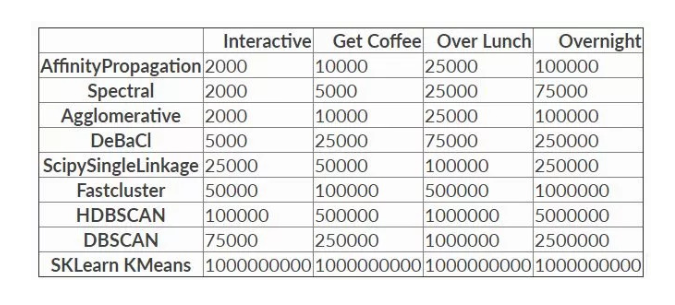因此不难看出，K均值算法最大的优点就是运行速度快，能够处理的数据量大，且易于理解。但缺点也很明显，就是算法性能有限，在高维上可能不是最佳选项。
一个比较粗浅的结论是，在数据量不大时，可以优先尝试其他算法。当数据量过大时，可以试试HDBSCAN。仅当数据量巨大，且无法降维或者降低数量时，再尝试使用K均值。
一个显著的问题信号是，如果多次运行K均值的结果都有很大差异，那么有很高的概率K均值不适合当前数据，要对结果谨慎的分析。
此外无监督聚类的评估往往不易，基本都是基于使用者的主观设计，如sklearn中提供的Silhouette Coefficient和 Calinski-Harabaz Index 。更多关于无监督学习如何评估可以参考 [微调：一个无监督学习算法，如何判断其好坏呢?]。
参考来源聚类分析（五）基于密度的聚类算法 — DBSCAN聚类算法第三篇-密度聚类算法DBSCAN聚类算法初探（五）DBSCAN，作者: peghoty聚类算法第一篇-概览sklearn.cluster.DBSCAN【挖掘模型】：Python-DBSCAN算法


展开全文class 算法 DBSCAN 聚类
• DPC数据库 基于密度骨干和模糊邻域的密度峰聚类 Abdulrahman Lotfi a ， Parham Moradi a ， ... 此策略可防止连锁React，并为位于边界区域的那些实例有效分配真实标签，以有效地聚类具有各种形状和密度的数据。 DPMATLAB
• 1. 对于空间中的一个对象，如果它在给定半径e的邻域中的对象个数大于密度阀值MinPts，则该对象被称为核心对象，否则称为边界对象。 2. 如果p是一个核心对象，q属于p的邻域，那么称p直接密度可达q。 3. 如果...

基于密度的聚类

定义：

1.      对于空间中的一个对象，如果它在给定半径e的邻域中的对象个数大于密度阀值MinPts，则该对象被称为核心对象，否则称为边界对象。

2.      如果p是一个核心对象，q属于p的邻域，那么称p直接密度可达q。

3.      如果存在一条链<p1,p2,…..,pi>，满足p1=p，pi=q，pi直接密度可达pi+1，则称p密度可达q。

4.      如果存在o，o密度可达q和p，则称p和q是密度连通的

5.      由一个核心对象和其密度可达的所有对象构成一个聚类。a为核心对象，b为边界对象，且a直接密度可达b，

但b不直接密度可达a,因为b不是一个核心对象c直接密度可达a,a直接密度可达b,所以c密度可达b，

同理b不密度可达c，但b和c密度连通

DBSCAN从任一对象p开始，根据参数e和MinPts提取所有从p密度可达对象，得到一个聚类。

1.      从任一对象p开始。

a)        如果p是核心对象，则p和p直接密度可达的所有对象被标记为类i。递归p直接密度可达的所有对象qi（即用qi代替p回到第一步）。

b)        如果p是一个边界对象，那么p被标记为噪声。

2.      i++

3.      如果还有没被标记的对象，则从中任选一个作为p，回到第一步。得到一个类，同样我们可以得到另一个类优点：

1.      对噪声不敏感。

2.      能发现任意形状的聚类。

缺点：

1.      聚类的结果与参数有很大的关系。

2.      DBSCAN用固定参数识别聚类，但当聚类的稀疏程度不同时，相同的判定标准可能会破坏聚类的自然结构，即较稀的聚类会被划分为多个类或密度较大且离得较近的类会被合并成一个聚类

附：

专题思路：把搜下来的网页进行聚类，将聚类结果显示给用户，用户可以选择其中的一个类，标为关注，类的关键词作为主题，用户就可以跟踪这主题、了解主题的文章的情感（就是其它部分的功能）


展开全文• 基于密度的算法 基于密度的算法的核心思想是根据样本点某一邻域内的邻居数定义样本空间的密度，这类算法可以...它将定义为样本点密集的区域，算法从一个种子样本开始，持续向密集的区域生长直至到达边界。 　算法使...
基于密度的算法
基于密度的算法的核心思想是根据样本点某一邻域内的邻居数定义样本空间的密度，这类算法可以找出空间中形状不规则的簇，并且不用指定簇的数量。算法的核心是计算每一点处的密度值，以及根据密度来定义簇。
1.DBSCAN算法理论
DBSCAN算法是一种基于密度的算法，可以有效的处理噪声，发现任意形状的簇。它将定义为样本点密集的区域，算法从一个种子样本开始，持续向密集的区域生长直至到达边界。
算法使用了两个人工设定的参数$\varepsilon$和$M$，前者是样本点的领域半径，后者是定义核心点的样本数阈值。下面介绍他们的概念。
假设有样本集$X=\{x_1,x_2,...,x_N\}$，样本点$x$的$\varepsilon$邻域定义为样本集中与该样本的距离小于等于$\varepsilon$的样本构成的集合
$N_\varepsilon(x)=\{y\in X:d(x,y)\}$
其中，$d(x,y)$是两个样本之间的距离，可以采用任何一种距离定义。样本的密度定义为它的$\varepsilon$邻域的样本数：
$\rho(x)=|N_\varepsilon(x)|$
密度是一个非负整数。核心点定义为数据集中密度大于指定阈值的样本点，即如果
$\rho(x)\ge M$
则称$x$为核心点，核心点是样本分布密集的区域。样本集$X$中所有的核心点构成的集合为$X_c$，非核心点构成的集合为$X_nc$。如果$x$是非核心点，并且它的$\varepsilon$邻域内存在核心点，则称$x$为边界点，边界点是密集区域的边界。如果一个点既不是核心点也不是边界点，则称之为噪声点，噪声点是样本稀疏的区域。
如果$x$是核心点，$y$在它的$\varepsilon$邻域内，则称$y$是从$x$直接密度可达的。对于样本集中 的一组样本$x_1,x_2,...,x_n$，如果$x_i$$_+$$_1$是从$x_i$直接密度可达的，则称$x_n$是从$x_i$可达的，密度可达是直接密度可达的推广。
对于样本集中的样本点$x,y$和$z$,如果$y$和$z$都从$x$密度可达，则称他们是密度相连的，根据定义，密度相连具有对称性。
基于上面的概念可以给出簇的定义。样本集$C$是整个样本集的一个子集，如果它满足下列条件：对于样本集$X$中的任意两个样本$x$和$y$，如果$x\in C$,且$y$是从$x$直接密度可达的，则$y\in C$；如果$x\in C,y\in C$，则$x$和$y$是密度相连的，则称集合$C$是一个簇。
根据簇的定义可以构造出聚类算法，具体做法是从某一核心点出发，不断   向密度可达的区域扩张，得到一个包含核心点和边界点的最大区域。这个区域中惹事两点的密度相连。
假设有样本集$X$,聚类算法将这些样本划分成$K$个簇以及噪声点的集合，其中$K$由算法确定。每个样本要么属于这些簇中的一个，要么是噪声点。定义变量$m_i$为样本$x_i$所属的簇，如果它属于第$j$个簇，则$m_i$的值为$j$,如果它不属于这些簇中的任意一个，即是噪声点，则其值为-1，$m_i$就是聚类算法的返回结果。变量$k$表示当前的簇号，每发现一个新的簇其值加1。聚类算法流程如下：
第一阶段，初始化
计算每个样本的邻域$N_\varepsilon(x_i)$
令$k=1,m_i=0$,初始化待处理样本集合$I=\{1,2,...,N\}$
第二阶段，生成所有的簇
循环，当$I$不为空
从$I$中取出一个样本$i$，并将其从集合中删除
如果$i$没被处理过，即$m_i=0$
初始化集合$T=N_\varepsilon(x_i)$
如果$i$为非核心点
令$m_i=-1$，暂时标记为噪声
如果$i$为核心点
令$m_i=k$，将当前簇编号赋予该样本
循环，当$T$不为空
从集合$T$中取出一个样本$j$并从该集合中将其删除
如果$m_i=0$或者$m_i=-1$
令$m_i=k$
如果$j$是核心点
将$j$的邻居集合$N_\varepsilon(x_i)$加入集合$T$
结束循环
令$k=k+1$
结束循环

算法的核心步骤是依次处理每一个还未标记额的点，如果是核心点，则将其邻居点加入到连接的结合浩总，反复扩张，直到找到一个完整的簇。
在实现时有几个问题需要考虑，第一个问题是如何快速找到一个点的所有邻居集合，可以用R树或者KD树等数据结构加速，第二个问题是参数$\varepsilon$和$M$的设定，$\varepsilon$的取值在有的时候非常难以确定，而它对聚类的结果有很大影响。$M$值的选择有一个指导性原则，如果样本向量是$n$维的，则$M$的取值至少为$n+1$。
DBSCAN无须指定簇的数量，可以发现任意形状的簇，并且对噪声不敏感。其缺点是聚类的质量受距离函数的影响很大，如果数据维度很高，将面临维度灾难的问题。参数$\varepsilon$和$M$的设定有时候很困难。
2.DBSCAN算法的sklearn实现
在sklearn库中包含着各种各样的聚类算法，下面我们实现DBSCAN算法并实现其他聚类算法与DBSCAN算法形成对照。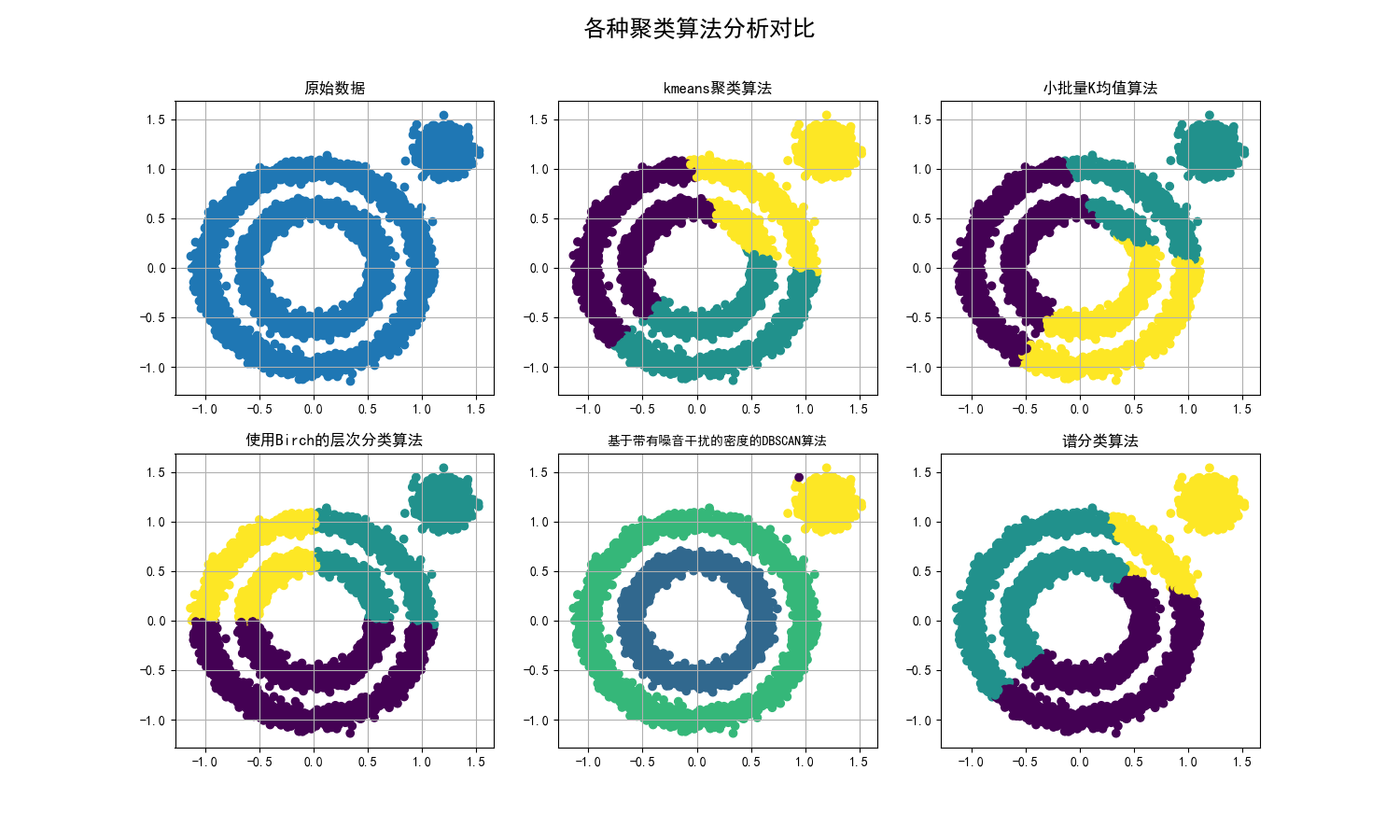五种算法代码实现
import numpy as np
import matplotlib.pyplot as plt
import matplotlib as mpl
from sklearn.cluster import KMeans
from sklearn import datasets
# 小批量K均值算法
from sklearn.cluster import MiniBatchKMeans
# 使用Birch的层次分类方法
from sklearn.cluster import Birch
# 基于带有噪音干扰的密度分类法
from sklearn.cluster import DBSCAN
# 谱分类，需要计算基于rbf距离的相似矩阵
from sklearn.cluster import SpectralClustering
x1, _ = datasets.make_circles(n_samples=5000, factor=.6, noise=0.05)
x2, _ = datasets.make_blobs(n_samples=1000, n_features=2, centers=[[1.2, 1.2]], cluster_std=[[.1]], random_state=9)
data = np.concatenate((x1, x2), axis=0)
plt.figure(figsize=(15, 9))
plt.subplot(231)
# 解决中文无法显示问题
mpl.rcParams['font.sans-serif'] = [u'SimHei']
mpl.rcParams['axes.unicode_minus'] = False
# 原始数据作图
plt.scatter(data[:, 0], data[:, 1])
plt.title("原始数据")
plt.grid(True)
plt.subplot(232)
#使用Kmeans聚类进行分析
result = KMeans(n_clusters=3, random_state=9).fit_predict(data)
plt.scatter(data[:, 0], data[:, 1], c=result)
plt.title("kmeans聚类算法")
plt.grid(True)
# 小批量K均值算法
plt.subplot(233)
result = MiniBatchKMeans(n_clusters=3, random_state=9).fit_predict(data)
plt.scatter(data[:, 0], data[:, 1], c=result)
plt.title("小批量K均值算法")
plt.grid(True)
# 使用Birch的层次分类方法
plt.subplot(234)
result = Birch(n_clusters=3).fit_predict(data)
plt.scatter(data[:, 0], data[:, 1], c=result)
plt.title("使用Birch的层次分类算法")
plt.grid(True)
# 基于带有噪音干扰的密度分类法,这种方法不需要制定分类类别，但是需要制定距离和每一簇的最小样本数。
plt.subplot(235)
result = DBSCAN(eps=0.1, min_samples=10).fit_predict(data)
plt.scatter(data[:, 0], data[:, 1], c=result)
plt.title("基于带有噪音干扰的密度的DBSCAN算法",fontsize=10)
plt.grid(True)
# 谱分类，需要计算基于rbf距离的相似矩阵
plt.subplot(236)
result = SpectralClustering(n_clusters=3).fit_predict(data)
plt.scatter(data[:, 0], data[:, 1], c=result)
plt.title("谱分类算法")#需要计算基于rbf距离的相似矩阵
plt.suptitle(u'各种聚类算法分析对比', fontsize=18)
plt.show()

请大家多多指教！
注：原理参考《机器学习与应用》雷明著，清华大学出版社


展开全文机器学习 聚类算法
• 在半径eps邻域内含有小于minpts数目的点，则为边界点，这些点都在核心的邻居。 噪声点 不是核心点和边界点的点 核心对象 如果对象的Eps邻域至少包含最小数目MinPts的对象，则称该对象为核心对象。 eps邻域 给定...
• Libagf是一个机器学习库，其中包括使用高斯核和k近邻的自适应核密度估计器。 操作包括统计分类，内插/非线性回归和pdf估计。 对于统计分类，有一个边界训练功能可用于创建快速且通用的预训练模型，但该模型仍返回...开源软件
• 首先，我们解决了在应用非参数方法估计违约回收率密度函数时如何选取窗宽，以及在使用对称时如何处理在有界区间上产 生的边界问题. 针对如何选取合理窗宽提高估计效果的问题，我们通过将最优窗宽选取通过渐近积分...
• 1）如果一个数据点周围足够稠密，也就是以这个点为中心，给定半径的邻域内的数据点足够多，密度大于密度阈值(用户指定的参数MinPts),则称这个数据点为核心数据点，否则为边界数据点。 2）研究数据点之间的关系，如果...
• 我们分析了格林函数，卡西米尔密度和与在高维宇宙弦时空中限制在两个平行板之间的大规模标量量子场相关的力。 板垂直于弦线放置，并且场服从其上的罗宾边界条件。 对于单板和两板几何形状，在场平方和能量动量张量的...
• 针对传统模糊聚类( KFCM)算法无法克服边界噪声数据影响且对初始聚类中心敏感的不足,提出一种基于样本密度和最大类间方差法相结合的 KFCM算法。该算法在传统的 KFCM算法中引入样本分布密度作为权重,克服噪声及边界...
• 在分析节点度以及节点与邻居节点的局部覆盖密度后提出核心节点评价模型，并在此基础上给出局部核心节点识别方法；基于局部核心节点，提出新的面向重叠社区的异步标签传播策略，该策略能够快速地识别出社区内部节点与...复杂网络 标签传播
• 1. 对于空间中的一个对象，如果它在给定半径e的邻域中的对象个数大于密度阀值MinPts，则该对象被称为核心对象，否则称为边界对象。 2. 如果p是一个核心对象，q属于p的邻域，那么称p直接密度可达q。 3. 如果...聚类 DBSCAN
• DBSCAN的核心思想是从某个核心点出发，不断向密度可达的区域扩张，从而得到一个包含核心点和边界点的最大化区域，区域中任意两点密度相连。 1、伪代码 算法： DBSCAN 输入： E — 半径 MinPts — 给定点在 E 领域内...DBSCAN python
• 在该算法中，通过高斯函数将原始样本空间中的点非线性变换到高维特征空间，以达到线性可聚的目的，并引入了一种基于局部密度的初始聚类中心选择方法，从而改善聚类效果。为了提高采样质量，结合划分后各类簇的样本...聚类分析 主动学习 分类
• Canvas ScalerCanvas Scaler是Unity UI系统中，控制UI元素的总体大小和像素密度的Compoent，Canvas Scaler的缩放比例影响著Canvas下的元素，包含字体大小和图像边界。SizeReference Resolution：预设萤幕大小Screen ...Unity3D UGUI
• 概念：基于密度的带有噪声点的聚类方法。 内部概念理解： 1.核心对象：若某个点的密度达到算法设定的阈值则称为核心点。 2.距离阈值：设定的半径r。 ...6.边界点：属于某个类的非核心点，不能发...DBSCAN
• 注意到有可能将压缩到较小的体积，并且使用RSC相比奈梅亨电势，变得更有边界。 还显示出奈梅亨的单个颗粒水平的光谱比压缩下的RSC电位增长更快。 最后，除内部区域外，径向密度分布保持恒定，而使用RSC时，其...
• 5边界点：如图中的B、C，如名字的直接含义 6离群点：如图中的N，如名字的直接含义 算法工作原理 假设密度阈值为4。 第一步点A半径为E的区域内有4个点达到了阈值。 第二步：以A点周边的点为对象，半径为E的区域画圆...机器学习
• DBSCAN基本概念： 邻域的距离阈值：设定的半径r 核心对象：若某个点的密度达到算法设定的阈值，则为核心点 直接密度可达：若点p在q的r领域内并且q是核心点，则p-q直接密度可达 密度可达：直接密度可达的...根据已有分类算法
• 文章目录@[toc]图形创建与保存创建保存图形参数符号和线条颜色文本属性...核密度图 箱线图 点图 散点图 折线图 相关图 马赛克图 添加图形内容 图形参数：符号、线条、颜色、文本属性、图形尺寸与边界尺寸 标题...R语言 可视化
• 利用程序估算-幔边界（尽管是固-液界面）处的这些物理量，假定下地幔p波速度为13.7km/s，S波速度为7.2km/s，密度为5.5g/cm³，地的P波速度为8.0km/s，S波速度为0.0km/s，密度为 9.9g/cm³。
• 利用程序估算-幔边界（尽管是固-液界面）处的这些物理量，假定下地幔p波速度为13.7km/s，S波速度为7.2km/s，密度为5.5g/cm³，地的P波速度为8.0km/s，S波速度为0.0km/s，密度为 9.9g/cm³。
• 基本名词 DBSCN 是根据密度分组的聚类算法 核心对象： 弱某个点的密度达到算法设定的阈值则这个点是核心点 ϵ\epsilonϵ- 邻域的距离阈值： 设定的...边界点： 有属于他的簇 但是没有下线的点 噪声点： 不属于任何一...